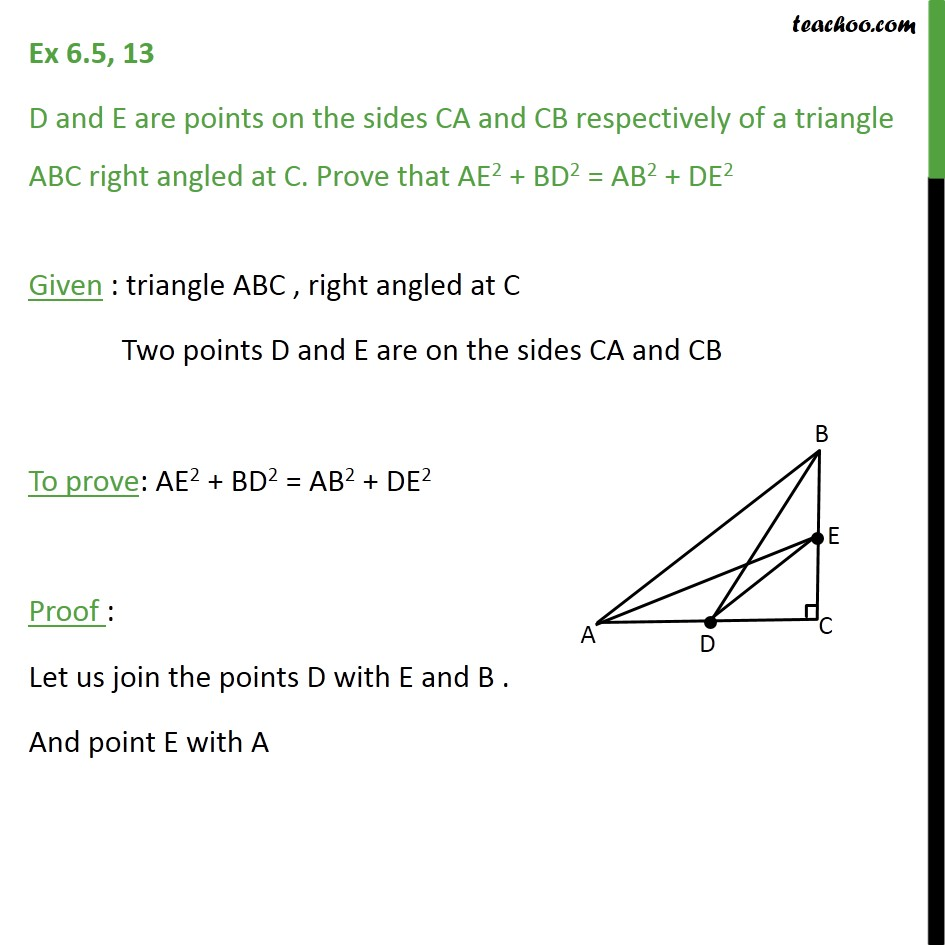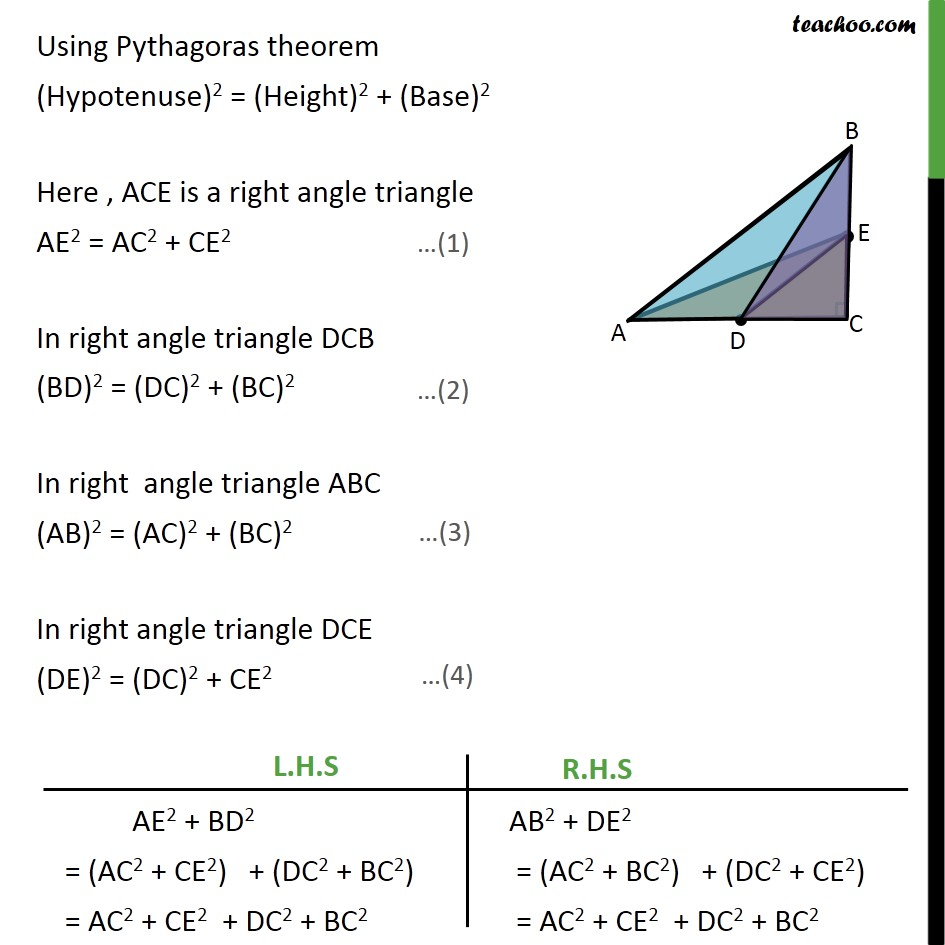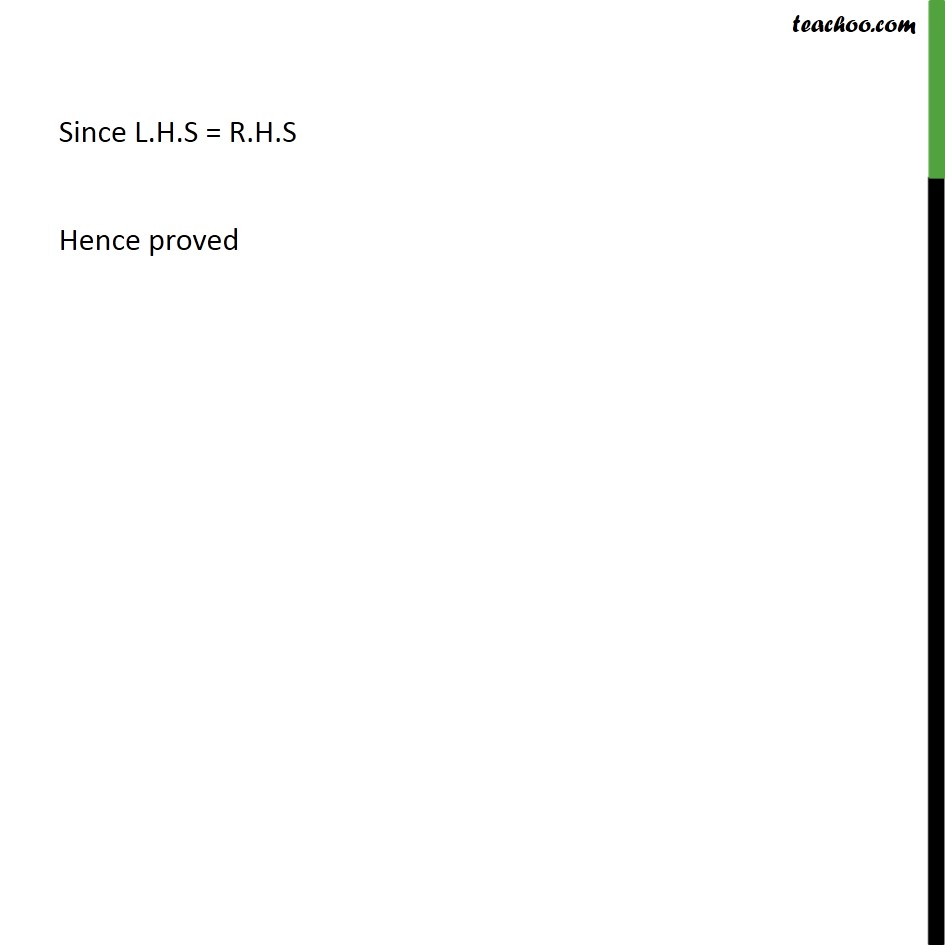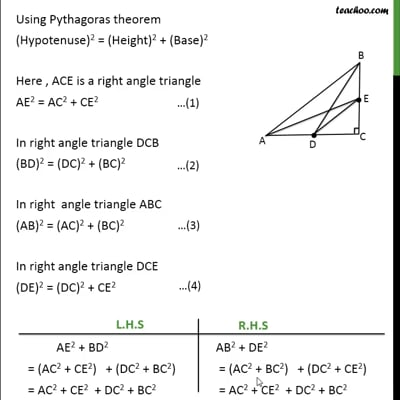Ex 6.5

Chapter 6 Class 10 Triangles
Serial order wiseThis video is only available for Teachoo black users

Solve all your doubts with Teachoo Black (new monthly pack available now!)

### Transcript

Ex 6.5, 13 D and E are points on the sides CA and CB respectively of a triangle ABC right angled at C. Prove that AE2 + BD2 = AB2 + DE2 Given : triangle ABC , right angled at C Two points D and E are on the sides CA and CB To prove: AE2 + BD2 = AB2 + DE2 Proof : Let us join the points D with E and B . And point E with A Using Pythagoras theorem (Hypotenuse)2 = (Height)2 + (Base)2 Here , ACE is a right angle triangle AE2 = AC2 + CE2 In right angle triangle DCB (BD)2 = (DC)2 + (BC)2 In right angle triangle ABC (AB)2 = (AC)2 + (BC)2 In right angle triangle DCE (DE)2 = (DC)2 + CE2 Since L.H.S = R.H.S Hence proved